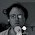## Friday, September 21, 2012

### Plotting Watts-Strogatz model

Recently I wanted to reproduce Figure 2 from Watts and Strogatz (1998). The task using igraph is simple but an interesting task was annotation of the resulting plot.

Watts-Strogatz model generates graphs that have so called small-world network property. Such networks should have low average path length and high clustering coefficient. The algorithm has three parameters: number of nodes in the graph, initial number of neighbors of each node distributed on a ring and rewiring probability.

Interestingly in Watts-Strogatz model having small but positive values of rewiring probability generates graphs having desired properties - and this is exactly depicted on Figure 2 in their article.

I decided to replicate it. To enhance it I wanted to plot median and 5 and 95 percentile of distribution of average path length and clustering coefficient as a function of rewiring probability.

Here you have the code that generates the graph (warning: it takes about 1 minute to run):

library(igraph)
set.seed(1)

avg.stat <- function(nei, p) {
result <- replicate(1000, {
wsg <- watts.strogatz.game(1, 100, nei, p)
c(average.path.length(wsg),
transitivity(wsg))
})
apply(result, 1, quantile, probs = c(0.5, 0.05, 0.95))
}

nei <- 6
p <- 2 ^ -seq(0, 10, len = 21)
result <- sapply(p, avg.stat, nei = nei)
result <- t(result / rep(avg.stat(nei, 0)[1,], each = 3))
par(mar=c(3.220.20.2), mgp=c(210))
matplot(p, result, type = "l", log = "x", xaxt = "n", ylab = "",
lty = rep(c(1,2,2),2), col=rep(c(1,2), each=3))
axis(1, at = 2 ^ -(0:10),
labels =  c(1, parse(text = paste(2, 1:10, sep = "^-",
collapse ";"))))
legend("bottomleft", c("average path length", "clustering coefficient"),
lty = 1, col = c(1, 2))

The result of the procedure is the following picture:

It looks very similar to what is shown in the article (apart from adding lines depicting 5 and 95 percentile of distributions of both graph characteristics).

However, the interesting part was to properly annotate X-axis on the plot. Of course you can use expression function to get it but then the problem is that you have to do it ten times. Interestingly parsing a string containing those ten expressions separated by semicolons works just as needed.

#### 3 comments:

1.Btw. there is a demo in igraph that plots exactly this (after calculating and plotting some other related quantities.) Type

library(igraph)
demo("smallworld") # or
igraphdemo("smallworld")

2.I also got similar results with a Python program I wrote. However, with both my graph and yours, the average path length does not drop off as dramatically as it does in the graph in the Watts-Strogatz paper. On their graph, L(p)/L(0) goes almost to 0. My results don't go anywhere near 0. They go to about 0.6, while yours goes to 0.5.

Did you wonder what might be the cause for that.

1.Probably the reason is initial graph size. In Watts-Strogatz paper it has 1000 vertices and 10 edges per vertex before randomization. In my code I use 100 and 12 respectively.